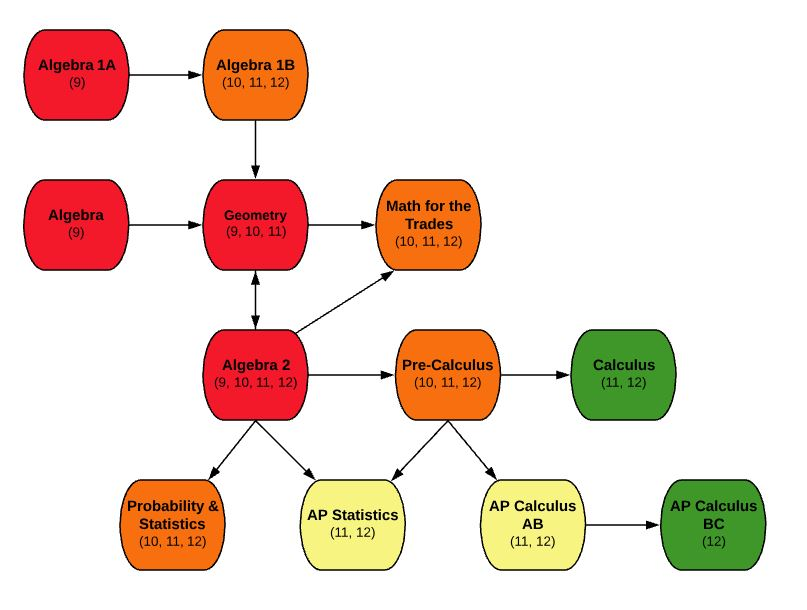EDIT MAIN

# MA200 Geometry9, 10, 11

Mathematics

Standard

1

This is a year long course that requires selection of both semesters: S1 and S2. The purpose of this course is to develop logic, measurement and problem solving skills necessary for continued study of mathematics. Students will develop the ability to find connections between physical shapes and algebra. An emphasis of this course is on Euclidean geometry, logic skills of problem solving, and applications of algebra.

Outcomes:

• Understand properties of parallel and perpendicular lines
• Understand properties of segments and angles
• Write and graph linear equations
• Prove triangles congruent and similar using appropriate theorems
• Understand properties of polygons
• Use trigonometry and special right triangles to solve triangles
• Understand vocabulary and equations of circles
• Calculate area and perimeter of 2-D shapes and surface area and volume of 3-D shapes

MA110-S1 – Algebra S1 AND MA110-S2 – Algebra S2
or
MA107-S1 – Algebra 1B S1 AND MA107-S1 – Algebra 1B S2

If you take this course, you must also take MA200-S2 – Geometry S2